# How To Divide Using Division Formula In Excel

This time we will venture how to divide division formulas in Excel; we will use examples, syntax, and figures to easy to understand and practice it your perspective.

Microsoft Excel provides different ways how to divide numbers and cells just like in any other basic math operator. It is in your hands what ways you will use considering your preference and requirements of your task. This is the continuation of our tutorial How To Multiply Cells In Excel With Examples.

Additionally, Microsoft Excel is a tool helpful in data entry, management of tasks, recording, and accounting stuff. Therefore a lot of business and work tasks use this application in organizing data and making financial analyses connecting to dividing a load task and finances.

Furthermore, if you use Excel thus encounter mathematical calculations such as division in your worksheet.

## What is the Division Formula in Excel

Particularly, Excel does not have a division formula or function, but there are a lot of alternatives we can use. Along with this, you can use an arithmetic operation symbol (/) or a quotient function. Later we will know the things to remember in creating a formula but first, let’s know the syntax…

## Syntax

`=number1 / number2`

It means that the` `=the number being divided / the number you are dividing by`.`

## Divide symbol

In a division in Excel, the common way to execute it using the division sign. Thus, in arithmetic operations, the division operator uses the obelus symbol (÷) while in excel it uses a forward slash (/) symbol representing it.

Wherefore the expression of this process is written like this =a/b with no spaces where:

• a is the dividend – a number you want to divide, and
• b is the divisor – a number by which the dividend is to be divided.

So here are a few reminders to be kept in mind in creating a formula. This will help you while we jump specifically into dividing in Excel.

1. First the equal sign(=). All the formulas must begin with this sign so that Excel will recognize them as formulas.
2. Use cell reference. This is where the result of the formula is displayed, not the actual formula. Thus, helpful to enter the data in the cell and if you need to go back to later values, it will be easier to understand.
3. Consider PEMDAS (Parenthesis, Exponentiation, Multiplication, Division, Addition, Subtraction) as a reference in the order of arithmetic operations. Below you’ll find an example of how the order of operations is applied to formulas in Excel.

## How to Divide in Excel

### Example 1: Using Numbers Directly

Here’s how to divide in Excel by typing numbers directly into the cell:

1. Click the cell where you want the result displayed.
•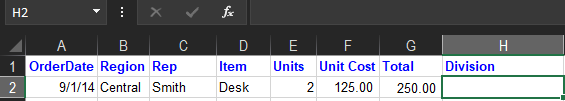2. Type the equal sign(=).
•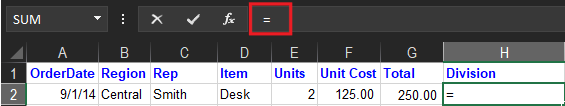3. Create your expression with the operator symbol (/). For instance, if you want to divide 20 by 10, enter 20/10 after the equal sign.
•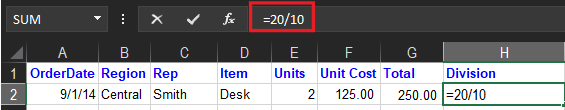4. When you press enter. In the example above, the result would be 2.
•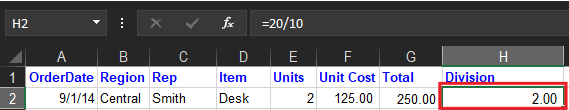Note: Importantly, type equal sign (=) in the cell before you type, the number and the / operator otherwise Excel will read it as date.

For example, if you type 11/10, Excel may display 10-Nov in the cell. Or, if you type 10/20, Excel will first convert that value to 10/30/2020 and display 20-Oct in the cell.

### Example 2: Using cell references

Here’s how to divide in Excel by using cell references instead of typing numbers directly into the cell:

1. Select the cell where you want the result to appear.
•2. Type the equal sign, and use cell references instead of typing regular numbers. For instance, if you want to divide cell E2 by cell G2, enter the formula =E2/G2.
•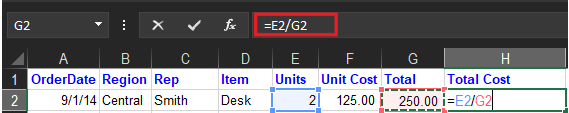3. When you press enter key, Excel displays the result in the cell.
•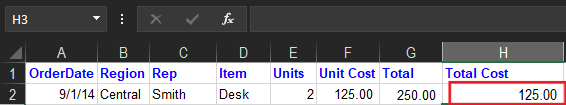## What is the QUOTIENT function in Excel?

The quotient Function is a formula where it divides the two numbers but returns the integer portion of a division. Moreover, it displays a whole number because it discards the rest. For instance, you want to have a quotient of 5 and 2 so the output is 2.5, hence it yields 2 only as the quotient only displays an integer.

## How to use the QUOTIENT function in Excel

So here are the steps on how to use QUOTIENT FUNCTION in Excel:

1. First, click the cell you want the result to display.
2. Enter the =QUOTIENT() into the cell.
3. After the parenthesis, type the first number, the dividend, followed by a comma. Then, enter the divisor which is the second number. Then, close the parenthesis.
4. It divides numbers in excel when you press enter.

Apparently, the value in the first number or numerator and denominator or second number for a quotient function could be a number, reference cell, cell, or both.

Examples of these are:

• =QUOTIENT(5,2)
• =QUOTIENT(A4,16)
• =QUOTIENT(B2:C3)

## Summary

In conclusion, learning how to divide in Excel is very helpful in complying with each task and requirement in business or on daily activities.